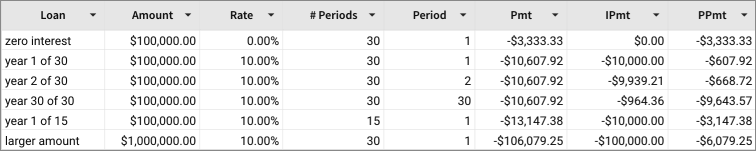# Pmt

The Pmt function returns the periodic payment for an investment.

You can use it to calculate constant payments for an investment or a loan, based on the loan amount, number of periods, and constant interest rate.

To determine how much of the payment is allocated to interest and how much to principal, use the IPmt and PPmt functions.

Pmt is part of the set of financial functions that Sigma supports.

## Syntax

`Pmt(rate, nperiods, pv, [fv], [type])`

The Pmt function has the following arguments:

rate
Required.
The interest rate for the loan.
nperiods
Required.
The total number of payments for the loan.
pv
Required.
The present value, or total value of all loan payments; the amount borrowed.
fv
Optional.
The future value, or a cash balance you want after the last payment is made.
Defaults to 0 (zero).
type
Optional.
When payments are due:
0
End of period
1
Beginning of period
Default is 0.

## Notes

• Be consistent with the units for rate and nperiods arguments. If you make monthly payments on a two-year loan at an annual interest rate of 7%, use the rate calculation of 0.07/12 and nperiods calculation of 2*12. For annual payments on the same loan, use the rate of 0.07 and nperiods of 2.
• Pmt returns the payment calculation that includes both the principal and interest portions. It does not include taxes, reserve payments, or fees.
• To calculate the total amount paid over the duration of the loan, multiply the returned Pmt value by nperiods.

## Examples

`Pmt(.07/12,2*12,10000)`

The monthly payment for a two-year loan of \$10,000, with an annual interest rate of 7% is \$447.73.

`Pmt(.07,2,10000)`

The annual payment for a two-year loan of \$10,000, with an annual interest rate of 7% is \$5,530.92.The yearly payment for a loan of \$100,000, with an annual interest rate of 10% over 30 years, compounded yearly, is \$10,607.92. Same conditions at half the time (15 years) result in a yearly payment of \$13,147.38.